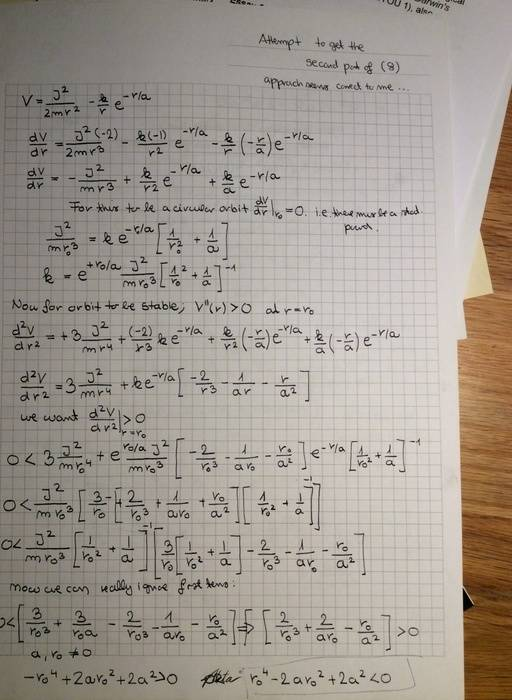# Stability of a circular orbit

## Homework Statement

In a classical model of a multi-electron atom, electrons are assumed to move in a modified electrostatic potential $V(r)$, given by;

$$V(r)=\dfrac{-k}{r}e^{-r/a}$$

Show that the effective potential is ;

$$V_e(r)=\dfrac{J^2}{2mr^2}+\dfrac{-k}{r}e^{-r/a}$$

Then show that the circular orbit is unstable unless;

$$0.5* (1+\sqrt{5}) \textgreater \dfrac{r}{a}$$

## Homework Equations

Take the derivative of the effective potential and using the fact that it is zero at the radius of the circular orbit, express the constant k.

Then take the second derivative and because the orbit is stable the stationary point, at the radius of the circular orbit, must be a minima, hence the second derivative evaluated at the point is greater than 0 for orbit to be stable.

You insert the k from line 1 in order to simplify the equation and some terms cancel.

## The Attempt at a Solution

I have tried to solve the problem multiple times and obtained;

$$k=e^{r/a}*\dfrac{J^2}{mr^3}(\dfrac{1}{r^2}+\dfrac{1}{a})^{-1}$$

this lead me to the inequality;

$$\sqrt{1+\sqrt{2}} \textgreater \dfrac{r}{a}$$

Could anyone tell me whether my approach is correct ??

Thank you

DEvens
Gold Member
What did you do to get that inequality?

What you were supposed to do, as the statement of the problem said, was use the expression for k to remove k from the potential. Then take the second derivative of that expression, now with no k in it. Then determine what makes that second derivative greater than 0.

What did you do?

That is exactly what I have done; I am attaching a figure with my working;.

DEvens
•